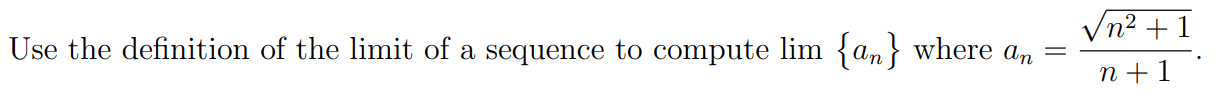# Limit of a Sequence (Updated with progress)

• MHB
slwarrior64Hello, I know I posted this question recently but I wanted to update with my progress. I have figured out what the limit should be but I would really appreciate help with how to use the definition of the limit of a sequence to prove it! What I have is:

Suppose n is extremely large, then both n^2+1 and n^2 have almost the same size. Similarly both n and n+1 have almost the same size. Therefore, as n grows larger and larger, we can replace the numerator by sqrt (n^2)=n and the denominator by n. Therefore the guess value for the limit should be 1 since the numerator and denominator should cancel.

I am pretty sure this is right, but I am not sure how to answer it in the way this question is asking.

Gold Member
MHB
View attachment 11131
Hello, I know I posted this question recently but I wanted to update with my progress. I have figured out what the limit should be but I would really appreciate help with how to use the definition of the limit of a sequence to prove it! What I have is:

Suppose n is extremely large, then both n^2+1 and n^2 have almost the same size. Similarly both n and n+1 have almost the same size. Therefore, as n grows larger and larger, we can replace the numerator by sqrt (n^2)=n and the denominator by n. Therefore the guess value for the limit should be 1 since the numerator and denominator should cancel.

I am pretty sure this is right, but I am not sure how to answer it in the way this question is asking.
That is definitely the right idea. The way to express it in more formal mathematical language is to divide top and bottom of the fraction by $n$ so as to get $$a_n = \frac{\sqrt{1 + \frac1{n^2}}}{1 + \frac1n}.$$ The numerator and denominator of that fraction both go to $1$ as $n\to\infty$, and you can use the theorem that the limit of a quotient is the quotient of the limits: $$\lim_{n\to\infty}\frac{\sqrt{1 + \frac1{n^2}}}{1 + \frac1n} = \frac{\lim_{n\to\infty}\sqrt{1 + \frac1{n^2}}}{\lim_{n\to\infty}\left(1 + \frac1n\right)} = \frac11 = 1.$$

slwarrior64
That is definitely the right idea. The way to express it in more formal mathematical language is to divide top and bottom of the fraction by $n$ so as to get $$a_n = \frac{\sqrt{1 + \frac1{n^2}}}{1 + \frac1n}.$$ The numerator and denominator of that fraction both go to $1$ as $n\to\infty$, and you can use the theorem that the limit of a quotient is the quotient of the limits: $$\lim_{n\to\infty}\frac{\sqrt{1 + \frac1{n^2}}}{1 + \frac1n} = \frac{\lim_{n\to\infty}\sqrt{1 + \frac1{n^2}}}{\lim_{n\to\infty}\left(1 + \frac1n\right)} = \frac11 = 1.$$

That makes sense, Thanks!